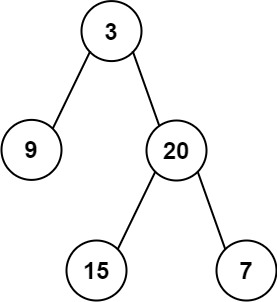105. Construct Binary Tree from Preorder and Inorder Traversal
Medium
12.5K
364

Given two integer arrays `preorder` and `inorder` where `preorder` is the preorder traversal of a binary tree and `inorder` is the inorder traversal of the same tree, construct and return the binary tree.

Example 1:```Input: preorder = [3,9,20,15,7], inorder = [9,3,15,20,7]
Output: [3,9,20,null,null,15,7]
```

Example 2:

```Input: preorder = [-1], inorder = [-1]
Output: [-1]
```

Constraints:

• `1 <= preorder.length <= 3000`
• `inorder.length == preorder.length`
• `-3000 <= preorder[i], inorder[i] <= 3000`
• `preorder` and `inorder` consist of unique values.
• Each value of `inorder` also appears in `preorder`.
• `preorder` is guaranteed to be the preorder traversal of the tree.
• `inorder` is guaranteed to be the inorder traversal of the tree.
Accepted
943.4K
Submissions
1.5M
Acceptance Rate
61.5%

Seen this question in a real interview before?
1/4
Yes
No

Discussion (0)

Related Topics